# Chapter 5 Regression 5192021 Chapter 5 1 Regression

• Slides: 20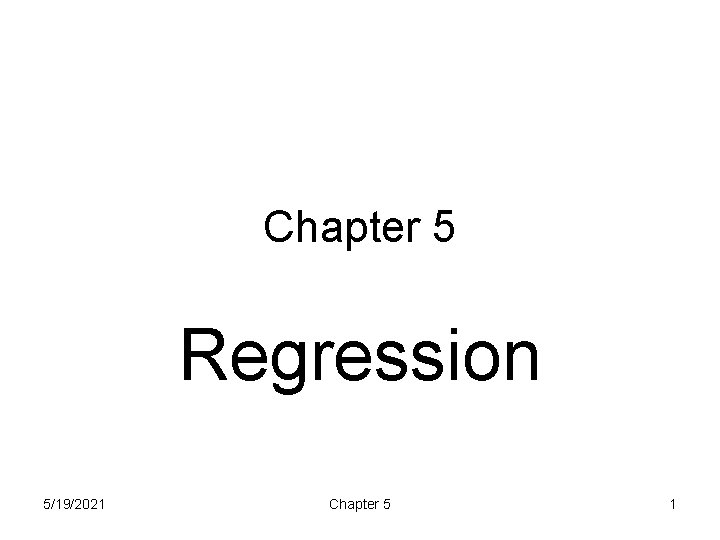Chapter 5 Regression 5/19/2021 Chapter 5 1Regression • Like correlation, regression addresses the relationship between a quantitative explanatory variable (X) and quantitative response variable (Y) • The objective of regression is to describe the best fitting line through the data • As with correlation, start by looking at the data with a scatterplot 5/19/2021 Chapter 5 2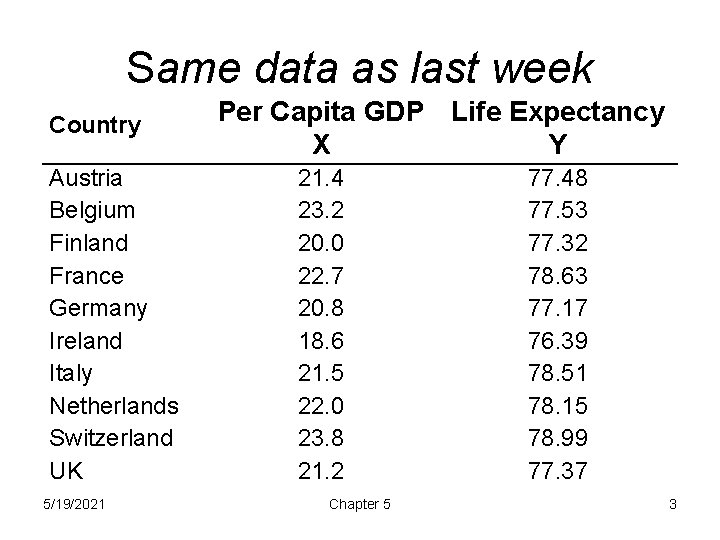Same data as last week Country Austria Belgium Finland France Germany Ireland Italy Netherlands Switzerland UK 5/19/2021 Per Capita GDP Life Expectancy X Y 21. 4 23. 2 20. 0 22. 7 20. 8 18. 6 21. 5 22. 0 23. 8 21. 2 Chapter 5 77. 48 77. 53 77. 32 78. 63 77. 17 76. 39 78. 51 78. 15 78. 99 77. 37 3Inspect scatterplot for linearity 5/19/2021 Chapter 5 4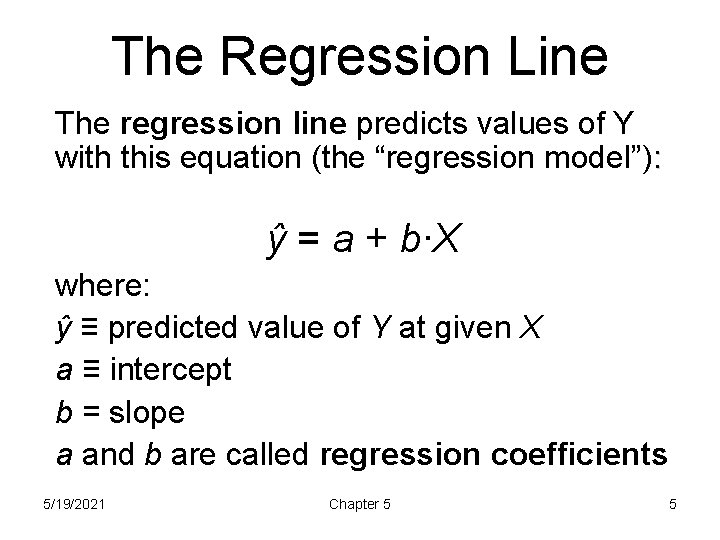The Regression Line The regression line predicts values of Y with this equation (the “regression model”): ŷ = a + b∙X where: ŷ ≡ predicted value of Y at given X a ≡ intercept b = slope a and b are called regression coefficients 5/19/2021 Chapter 5 5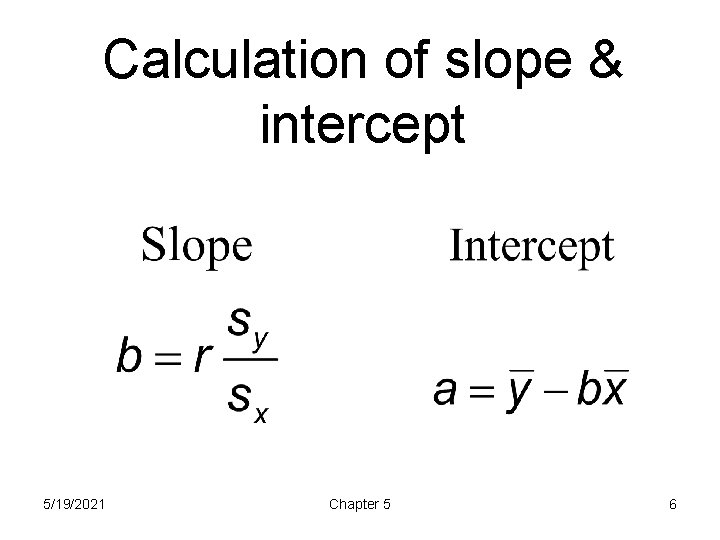Calculation of slope & intercept 5/19/2021 Chapter 5 6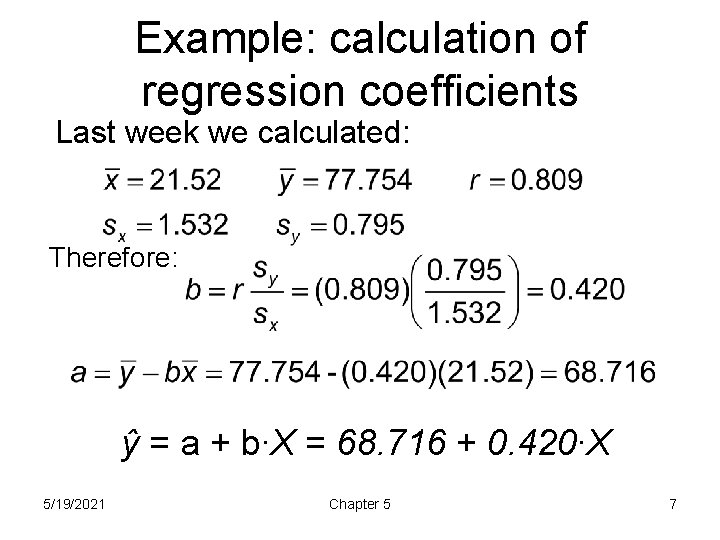Example: calculation of regression coefficients Last week we calculated: Therefore: ŷ = a + b∙X = 68. 716 + 0. 420∙X 5/19/2021 Chapter 5 7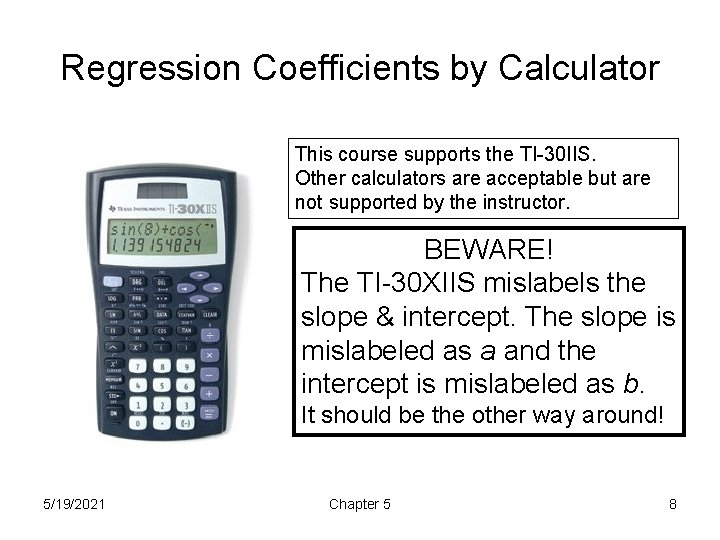Regression Coefficients by Calculator This course supports the TI-30 IIS. Other calculators are acceptable but are not supported by the instructor. BEWARE! The TI-30 XIIS mislabels the slope & intercept. The slope is mislabeled as a and the intercept is mislabeled as b. It should be the other way around! 5/19/2021 Chapter 5 8Interpretation of Slope b • The slope predicts the increase in Y per unit X. • Example: ŷ = 68. 7 + 0. 42∙X • The slope = 0. 42 Each unit increase in X (GDP) is associated with a 0. 420 increase in Y (life expectancy) 5/19/2021 Chapter 5 9Interpretation: Intercept a • The intercept is where the line would pass through the Y-axis (when X = 0). • Example: ŷ = 68. 7 + 0. 42∙X • The intercept = 68. 7. • We do NOT normally interpolate the intercept 5/19/2021 Chapter 5 10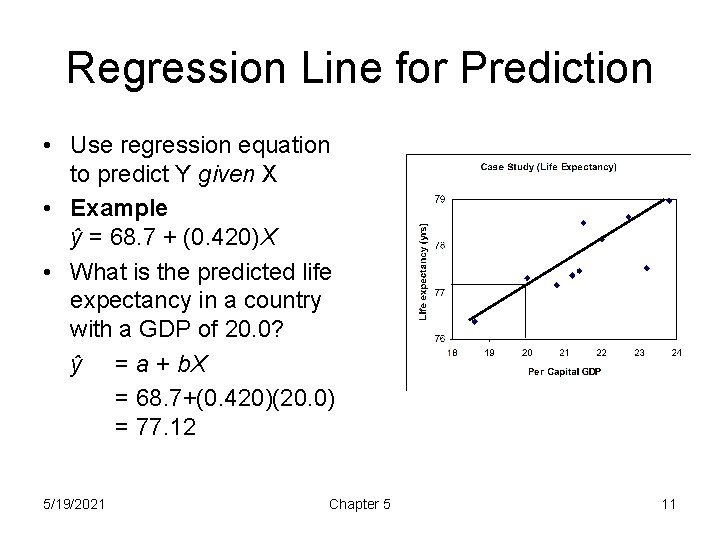Regression Line for Prediction • Use regression equation to predict Y given X • Example ŷ = 68. 7 + (0. 420)X • What is the predicted life expectancy in a country with a GDP of 20. 0? ŷ = a + b. X = 68. 7+(0. 420)(20. 0) = 77. 12 5/19/2021 Chapter 5 11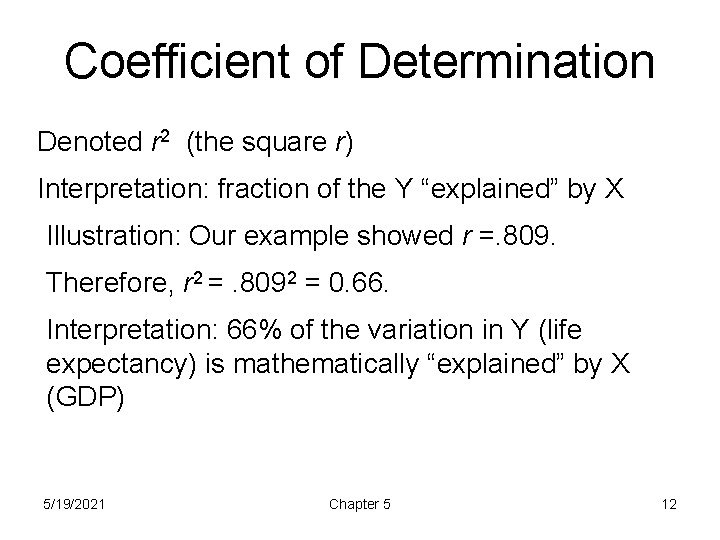Coefficient of Determination Denoted r 2 (the square r) Interpretation: fraction of the Y “explained” by X Illustration: Our example showed r =. 809. Therefore, r 2 =. 8092 = 0. 66. Interpretation: 66% of the variation in Y (life expectancy) is mathematically “explained” by X (GDP) 5/19/2021 Chapter 5 12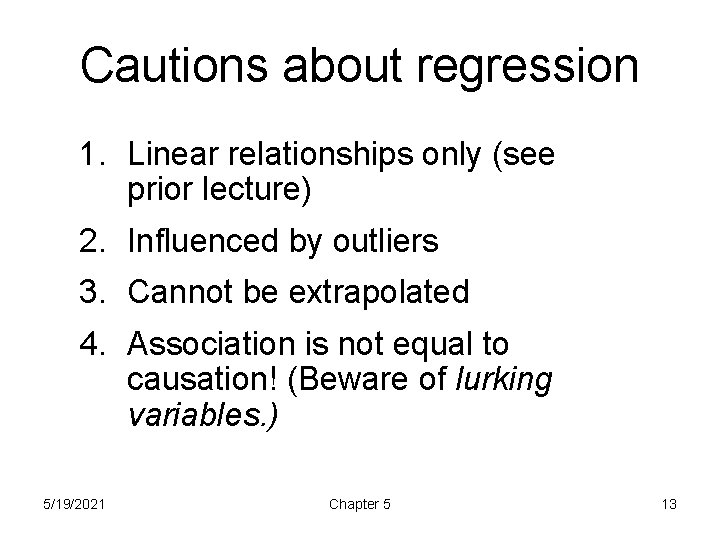Cautions about regression 1. Linear relationships only (see prior lecture) 2. Influenced by outliers 3. Cannot be extrapolated 4. Association is not equal to causation! (Beware of lurking variables. ) 5/19/2021 Chapter 5 13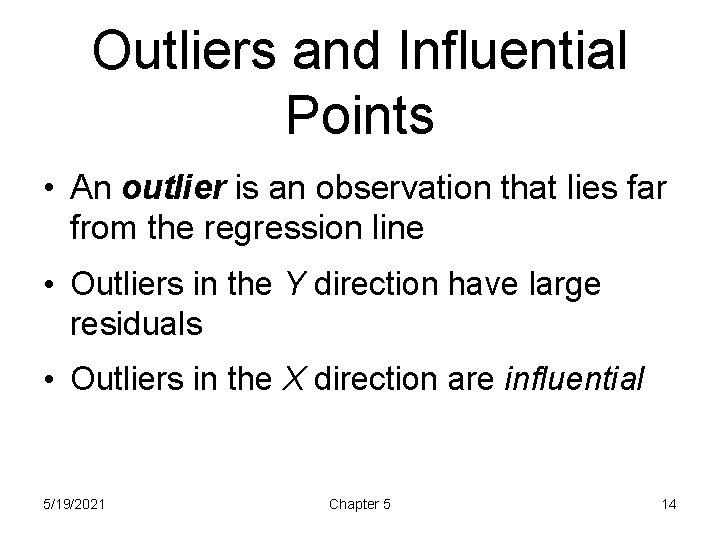Outliers and Influential Points • An outlier is an observation that lies far from the regression line • Outliers in the Y direction have large residuals • Outliers in the X direction are influential 5/19/2021 Chapter 5 14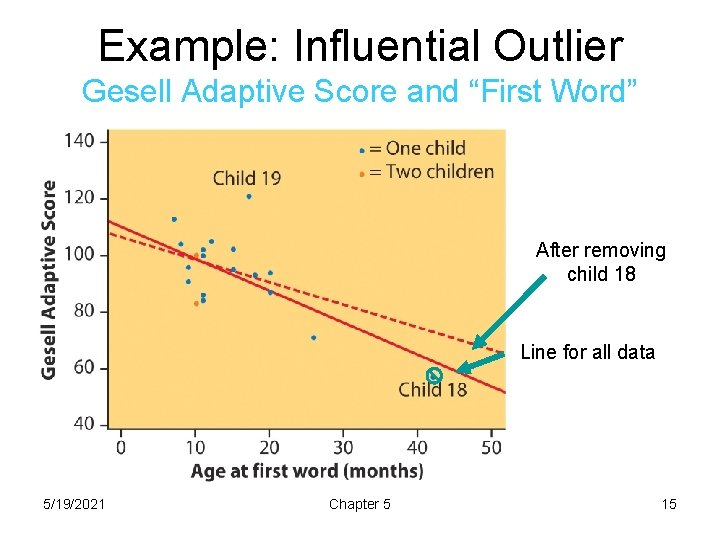Example: Influential Outlier Gesell Adaptive Score and “First Word” After removing child 18 Line for all data 5/19/2021 Chapter 5 15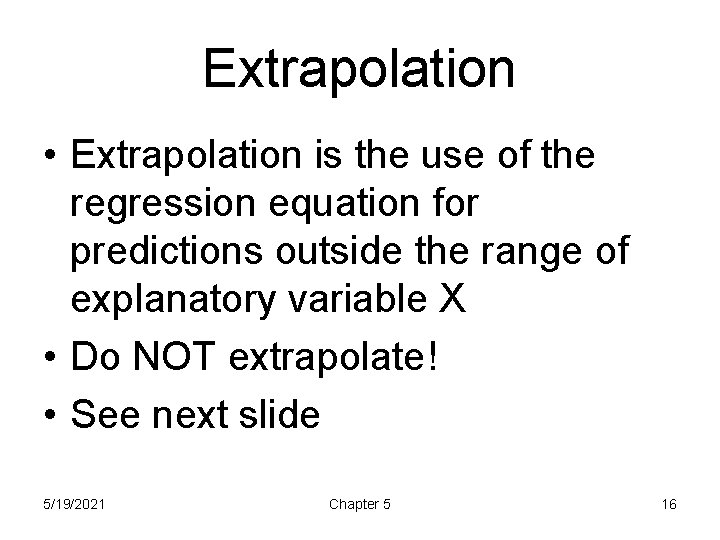Extrapolation • Extrapolation is the use of the regression equation for predictions outside the range of explanatory variable X • Do NOT extrapolate! • See next slide 5/19/2021 Chapter 5 16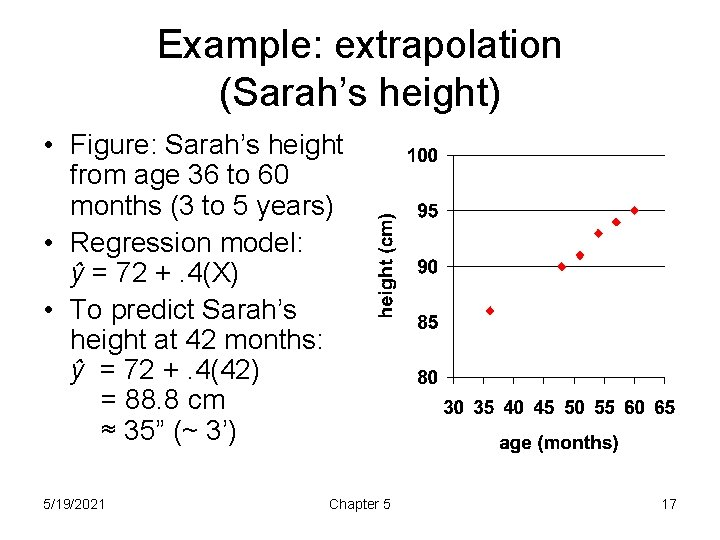Example: extrapolation (Sarah’s height) • Figure: Sarah’s height from age 36 to 60 months (3 to 5 years) • Regression model: ŷ = 72 +. 4(X) • To predict Sarah’s height at 42 months: ŷ = 72 +. 4(42) = 88. 8 cm ≈ 35” (~ 3’) 5/19/2021 Chapter 5 17Example: Extrapolation • Do NOT use the regression model to predict Sarah’s height at age 360 months (30 years)! • ŷ = 72 +. 4(X) = 72 +. 4(360) = 216 cm = more than 7’ tall (clearly ridiculous) 5/19/2021 Chapter 5 18Association does not imply causation Even strong correlations may be non-causal See pp. 144 – 145 for examples! 5/19/2021 Chapter 5 19Association does not imply causation Criteria to establish causation (pp. 144 – 146): • Strength of relationship • Experimentation • Consistency • Dose-response • Temporality • Plausibility 5/19/2021 Chapter 5 20